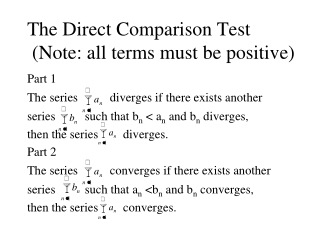DownloadDownload PresentationThe Direct Comparison Test (Note: all terms must be positive)

# The Direct Comparison Test (Note: all terms must be positive)

Télécharger la présentation## The Direct Comparison Test (Note: all terms must be positive)

- - - - - - - - - - - - - - - - - - - - - - - - - - - E N D - - - - - - - - - - - - - - - - - - - - - - - - - - -
##### Presentation Transcript

1. The Direct Comparison Test (Note: all terms must be positive) Part 1 The seriesdiverges if there exists another seriessuch that bn < an and bn diverges, then the series diverges. Part 2 The seriesconverges if there exists another seriessuch that an <bn and bn converges, then the series converges.

2. Example: Does the series converge?

3. If , then both and converge or both diverge. Limit Comparison Test If and for all (N a positive integer) Note: To find an appropriate seies to compare with, consider leading terms of numerator and denominator of given series.

4. Example: Does the series converge?